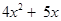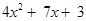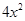### Algebraic Expressions - Revision Notes

CBSE Class–VII Subject Mathematics
Revision Notes

Chapter –12
Algebraic Expressions

• Algebraic expressions are formed from variables and constants. We use the operations of addition, subtraction, multiplication and division on the variables and constants to form expressions. For example, the expression
4xy + 7 is formed from the variables and and constants 4 and 7. The constant 4 and the variables and are multiplied to give the product 4xy and the constant 7 is added to this product to give the expression.
• Variable: Symbols which are used to represent or replace numbers. They are denoted as x, y, z, a, b, c…… and can take different numerical values. We generally use small letters to represent variables.
• Constant: A symbol having a fixed numerical value. Example: 2, -10, etc.
• Expressions are made up of terms. Terms are added to make an expression. For example, the addition of the terms 4xy and 7 gives the expression 4xy + 7.
• A term is a product of factors. The term 4xy in the expression 4xy + 7 is a product of factors xand 4. Factors containing variables are said to be algebraic factors.
• The coefficient is the numerical factor in the term. Sometimes anyone factor in a term is called the coefficient of the remaining part of the term.
• Any expression with one or more terms is called a polynomial.
• Specifically a one term expression is called a monomial.
• A two-term expression is called a binomial.
• A three-term expression is called a trinomial.
• Terms which have the same algebraic factors are liketerms. Terms which have different algebraic factors are unlike terms. Thus, terms 4xy and – 3xy are like terms; but terms 4xy and – 3are not like terms.
• The sum (or differenceof two like terms is a like term with coefficient equal to the sum (or differenceof the coefficients of the two like terms. Thus,
8xy – 3xy = (8 – 3 )xy, i.e., 5xy.
• When we add two algebraic expressions, the like terms are added as given above; the unlike terms are left as they are. Thus, the sum ofand
2+ 3 is; the like terms 5and 2add to 7x; the unlike termsand 3 are left as they are.
• In situations such as solving an equation and using a formula, we have to find the value of an expression. The value of the expression depends on the value of the variable from which the expression is formed. Thus, the value of 7– 3 for = 5 is 32, since 7(5) – 3 = 35 – 3 = 32.
• Rules and formulas in mathematics are written in a concise and general form using algebraic expressions: Thus, the area of rectangle = lb, where is the length and is the breadth of the rectangle.
• The general (nth) term of a number pattern (or a sequence) is an expression in n.
• Thus, the nth term of the number pattern 11, 21, 31, 41, . . . is (10+ 1).